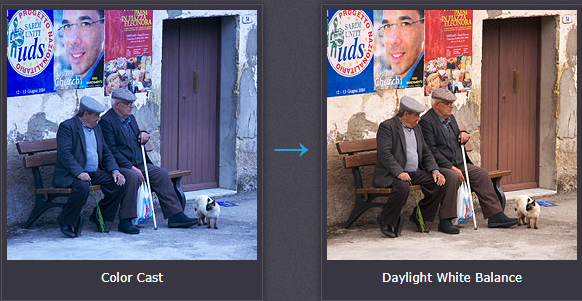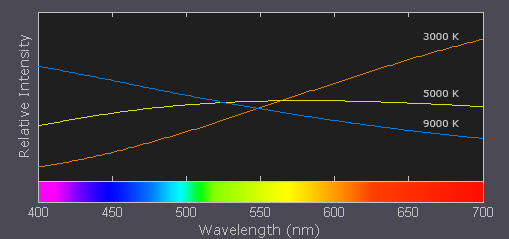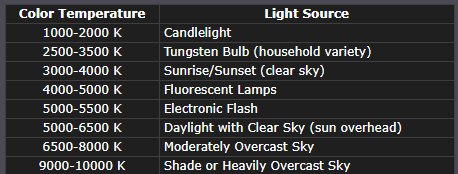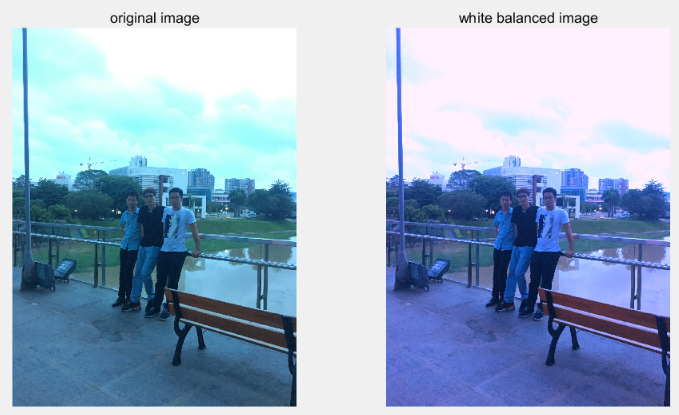# 图像处理中的白平衡

## 图像处理 之 白平衡

2012-11-03 10:30:00 weixin_34190136 阅读数 90
• ###### 自动白平衡

通过本课程的学习，可以对数字成像系统知识整体上得到理解，在相机等成像产品的开发，获得理论与实际的帮助。

822人学习 姜卓
免费试看## 图片白平衡处理方法

2018-10-18 11:28:46 mzl_18353516147 阅读数 1041
• ###### 自动白平衡

通过本课程的学习，可以对数字成像系统知识整体上得到理解，在相机等成像产品的开发，获得理论与实际的帮助。

822人学习 姜卓
免费试看

clc;
clear;
AveR=mean(mean(img(:,:,1)));  %获取R通道像素均值
AveG=mean(mean(img(:,:,2)));  %获取G通道像素均值
AveB=mean(mean(img(:,:,3)));  %获取通道像素均值
Qr=129/AveR;   %求取R通道系数
Qg=129/AveG;   %求取G通道系数
Qb=129/AveB;   %求取B通道系数
img0(:,:,1)=Qr*img(:,:,1); %求取变换后的R通道图像
img0(:,:,2)=Qg*img(:,:,2);%求取变换后的G通道图像
img0(:,:,3)=Qb*img(:,:,3);%求取变换后的B通道图像
figure,imshow(img),title('原图像');
figure,imshow(img0),title('白平衡变化后的图像');

原图像变换后的图像## 图像处理——白平衡（灰度世界方法）

2012-02-23 10:19:05 hellmonky 阅读数 6340
• ###### 自动白平衡

通过本课程的学习，可以对数字成像系统知识整体上得到理解，在相机等成像产品的开发，获得理论与实际的帮助。

822人学习 姜卓
免费试看

```im = imread('1.jpg','jpg');
im = double(im)./256;
r = im(:,:,1);
g = im(:,:,2);
b = im(:,:,3);
avgR = mean(mean(r));
avgG = mean(mean(g));
avgB = mean(mean(b));
avgGray = (avgR + avgG + avgB)/3;
if avgR == 0
outR = r;
else
outR = (avgGray/avgR).*r;
end

if avgG == 0
outG = g;
else
outG = (avgGray/avgG).*g;
end

if avgB == 0
outB = b;
else
outB = (avgGray/avgB).*b;
end

maxRGB = [max(max(outR)) max(max(outG)) max(max(outG))];

factor = max(maxRGB);
if  factor > 1
outR = outR./factor;
outG = outG./factor;
outB = outB./factor;
end

GWoutIm(:,:,1) = outR;
GWoutIm(:,:,2) = outG;
GWoutIm(:,:,3) = outB;

imshow(GWoutIm);

imwrite(GWoutIm, 'testout.jpg', 'jpg');

avgIn1RGB = [mean(mean(r)) mean(mean(g)) mean(mean(b))];
avgGW1RGB = [mean(mean(outR)) mean(mean(outG)) mean(mean(outB))];
avgIn1 = mean([mean(mean(r)) mean(mean(g)) mean(mean(b))]);
avgGW1 = mean([mean(mean(outR)) mean(mean(outG)) mean(mean(outB))]);```## MATLAB图像处理_图像的白平衡算法(灰色世界法)

2015-01-19 09:05:11 scottly1 阅读数 9381
• ###### 自动白平衡

通过本课程的学习，可以对数字成像系统知识整体上得到理解，在相机等成像产品的开发，获得理论与实际的帮助。

822人学习 姜卓
免费试看

```RGB=imread('test.jpg');

R = RGB(:,:,1);      G = RGB(:,:,2);      B = RGB(:,:,3);
Rx4 = RGB(:,:,1)*4;  Gx4 = RGB(:,:,2)*4;  Bx4 = RGB(:,:,3)*4; //Rx4只是做了一个简单的线性的提亮，可以不用参考。

Rave = mean(mean(R));
Gave = mean(mean(G));
Bave = mean(mean(B));
Kave = (Rave + Gave + Bave) / 3;

R1 = (Kave/Rave)*R; G1 = (Kave/Gave)*G; B1 = (Kave/Bave)*B;
R2 = (Kave/Rave)*Rx4; G2 = (Kave/Gave)*Gx4; B2 = (Kave/Bave)*Bx4;

RGB_white = cat(3, R1, G1, B1);
RGB_whitex4 = cat(3, R2, G2, B2);

RGB_white_out = uint8(RGB_white); RGB_white_outx4 = uint8(RGB_whitex4);
```## 图像白平衡原理及实现

2017-10-16 23:10:09 helimin12345 阅读数 9283
• ###### 自动白平衡

通过本课程的学习，可以对数字成像系统知识整体上得到理解，在相机等成像产品的开发，获得理论与实际的帮助。

822人学习 姜卓
免费试看

``````%%白平衡与色温紧密相关，不同色温光源下图像会呈现不同程度的偏色
%%由于人眼独特的适应性，在不同光照条件下观看物体时不会出现偏色，而就没这么先进了
%%蓝色光色温高，红色光色温低

clc;
clear all;
close all;
tic;
imgDst = imgSrc;
%%第一步，计算三个通道的平均灰度
imgR = imgSrc(:,:,1);
imgG = imgSrc(:,:,2);
imgB = imgSrc(:,:,3);
RAve = mean2(imgR);
GAve = mean2(imgG);
BAve = mean2(imgB);
aveGray = (RAve + GAve + BAve) / 3;
%%第二步，计算三个通道的增益系数
RCoef = aveGray / RAve;
GCoef = aveGray / GAve;
BCoef = aveGray / BAve;
%%第三步，使用增益系数来调整原始图像
RCorrection = RCoef * imgR;
GCorrection = GCoef * imgG;
BCorrection = BCoef * imgB;
imgDst(:,:,1) = RCorrection;
imgDst(:,:,2) = GCorrection;
imgDst(:,:,3) = BCorrection;
figure,subplot(1,2,1),imshow(imgSrc),title('original image');
subplot(1,2,2),imshow(imgDst),title('white balanced image');
toc;``````1. 白平衡与色温1. 白平衡的方法介绍

1. 计算三个通道的平均灰度
2. 计算三个通道的增益系数
3. 原始值乘上增益系数

matlab代码如下：1. 考虑硬件化实现

1. 帧有效期间，完成各个分量数据的累加；
2. 帧消隐期间，完成通道平均灰度与增益系数的计算；
3. 下一帧有效期间，使用上一帧计算的增益系数完成数据调整，同时，完成各个分量数据的累加，依此下去。